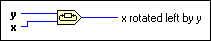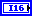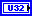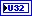# Rotate Function

## LabVIEW 2018 Help

Edition Date: March 2018
Part Number: 371361R-01
View Product InfoLabVIEW 2016 HelpLabVIEW 2017 HelpLabVIEW 2018 HelpLabVIEW 2019 HelpLabVIEW 2020 Help

Owning Palette: Data Manipulation Functions

Requires: Base Development System

Rotates x the number of bits specified by y.

The connector pane displays the default data types for this polymorphic function.y specifies the number of bits in x that the function rotates. If y is greater than 0, the function shifts the first y bits at the left end of x to the right end. If y is less than 0, the function shifts the first y bits at the right end of x to the left end.If x is an 8-, 16-, 32-, or 64-bit integer, then for any value of y, y ± 8, y ± 16, y ± 32, or y ± 64 yields the same output value, respectively, as y. For example, if x is an 8-bit integer, y = 1 and y = 9 yield the same result.x rotated left by y is the result of the rotation. The data type of x rotated left by y is determined by the data type of the x input.

## Rotate Details

The following table illustrates how x and y affect x rotated left by y: